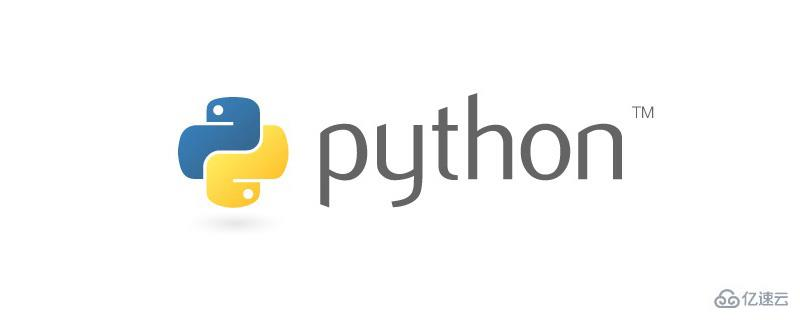# Python如何利用contextvars实现管理上下文变量Python 在 3.7 的时候引入了一个模块：contextvars，从名字上很容易看出它指的是上下文变量（Context Variables），所以在介绍 contextvars 之前我们需要先了解一下什么是上下文（Context）。

Context 是一个包含了相关信息内容的对象，举个例子："比如一部 13 集的动漫，你直接点进第八集，看到女主角在男主角面前流泪了"。相信此时你是不知道为什么女主角会流泪的，因为你没有看前面几集的内容，缺失了相关的上下文信息。

### web 框架中的 request

```# fastapi
from fastapi import FastAPI, Request
import uvicorn

app = FastAPI()

@app.get("/index")
async def index(request: Request):
name = request.query_params.get("name")
return {"name": name}

uvicorn.run("__main__:app", host="127.0.0.1", port=5555)

# -------------------------------------------------------

# sanic
from sanic import Sanic
from sanic.request import Request
from sanic import response

app = Sanic("sanic")

@app.get("/index")
async def index(request: Request):
name = request.args.get("name")
return response.json({"name": name})

app.run(host="127.0.0.1", port=6666)```

```from flask import Flask, request

@app.route("/index")
def index():
name = request.args.get("name")
return {"name": name}

app.run(host="127.0.0.1", port=7777)```

```import threading

# 创建一个 local 对象

def get():
# 获取绑定在 local 上的 value
value = local.value
print(f"线程: {name}, value: {value}")

def set_():
# 为不同的线程设置不同的值
if name == "one":
local.value = "ONE"
elif name == "two":
local.value = "TWO"
# 执行 get 函数
get()

t1.start()
t2.start()
"""

"""```

```{
"one": {"value": "ONE"},
"two": {"value": "TWO"}
}```

```# flask/globals.py
_request_ctx_stack = LocalStack()
_app_ctx_stack = LocalStack()
current_app = LocalProxy(_find_app)
request = LocalProxy(partial(_lookup_req_object, "request"))
session = LocalProxy(partial(_lookup_req_object, "session"))```

```# werkzeug/local.py

class Local(object):
__slots__ = ("__storage__", "__ident_func__")

def __init__(self):
# 内部有两个成员：__storage__ 是一个字典，值就存在这里面
# __ident_func__ 只需要知道它是用来获取线程 id 的即可
object.__setattr__(self, "__storage__", {})
object.__setattr__(self, "__ident_func__", get_ident)

def __call__(self, proxy):
"""Create a proxy for a name."""
return LocalProxy(self, proxy)

def __release_local__(self):
self.__storage__.pop(self.__ident_func__(), None)

def __getattr__(self, name):
try:
# 根据线程 id 得到 value（一个字典）
# 然后再根据 name 获取对应的值
# 所以只会获取绑定在当前线程上的值
return self.__storage__[self.__ident_func__()][name]
except KeyError:
raise AttributeError(name)

def __setattr__(self, name, value):
ident = self.__ident_func__()
storage = self.__storage__
try:
# 将线程 id 作为 key，然后将值设置在对应的字典中
# 所以只会将值设置在当前的线程中
storage[ident][name] = value
except KeyError:
storage[ident] = {name: value}

def __delattr__(self, name):
# 删除逻辑也很简单
try:
del self.__storage__[self.__ident_func__()][name]
except KeyError:
raise AttributeError(name)```

### contextvars

```import asyncio
import contextvars

c = contextvars.ContextVar("只是一个标识, 用于调试")

async def get():
# 获取值
return c.get() + "~~~"

async def set_(val):
# 设置值
c.set(val)
print(await get())

async def main():
coro1 = set_("协程1")
coro2 = set_("协程2")
await asyncio.gather(coro1, coro2)

asyncio.run(main())
"""

"""```

ContextVar 提供了两个方法，分别是 get 和 set，用于获取值和设置值。我们看到效果和 ThreadingLocal 类似，数据在协程之间是隔离的，不会受到彼此的影响。

```import asyncio
import contextvars

c = contextvars.ContextVar("只是一个标识, 用于调试")

async def get1():
return await get2()

async def get2():
return c.get() + "~~~"

async def set_(val):
# 设置值
c.set(val)
print(await get1())
print(await get2())

async def main():
coro1 = set_("协程1")
coro2 = set_("协程2")
await asyncio.gather(coro1, coro2)

asyncio.run(main())
"""

"""```

```import asyncio
import contextvars

c = contextvars.ContextVar("只是一个标识, 用于调试")

async def get1():
c.set("重新设置")
return await get2()

async def get2():
return c.get() + "~~~"

async def set_(val):
# 设置值
c.set(val)
print("------------")
print(await get2())
print(await get1())
print(await get2())
print("------------")

async def main():
coro1 = set_("协程1")
coro2 = set_("协程2")
await asyncio.gather(coro1, coro2)

asyncio.run(main())
"""
------------

------------
------------

------------
"""```

```import asyncio
import contextvars

c = contextvars.ContextVar("只是一个标识, 用于调试")

async def get1():
return await get2()

async def get2():
val = c.get() + "~~~"
c.set("重新设置啦")
return val

async def set_(val):
# 设置值
c.set(val)
print(await get1())
print(c.get())

async def main():
coro = set_("古明地觉")
await coro

asyncio.run(main())
"""

"""```

await get1() 的时候会执行 await get2()，然后在里面拿到 c.set 设置的值，打印 "古明地觉~~~"。但是在 get2 里面，又将值重新设置了，所以第二个 print 打印的就是新设置的值。\

```import asyncio
import contextvars

c = contextvars.ContextVar("只是一个标识, 用于调试",
default="哼哼")

async def set_(val):
print(c.get())
c.set(val)
print(c.get())

async def main():
coro = set_("古明地觉")
await coro

asyncio.run(main())
"""

"""```

```import asyncio
import contextvars

c = contextvars.ContextVar("只是一个标识, 用于调试",
default="哼哼")

async def set_(val):
print(c.get("古明地恋"))
c.set(val)
print(c.get())

async def main():
coro = set_("古明地觉")
await coro

asyncio.run(main())
"""

"""```

• 当 ContextVar 和 get 中都没有指定默认值，会抛出 LookupError；

• 只要有一方设置了，那么会得到默认值；

• 如果都设置了，那么以 get 为准；

```import threading
import contextvars

c = contextvars.ContextVar("context_var")

def get():
value = c.get()
print(f"线程 {name}, value: {value}")

def set_():
if name == "one":
c.set("ONE")
elif name == "two":
c.set("TWO")
get()

t1.start()
t2.start()
"""

"""```

### c.Token

```import contextvars

c = contextvars.ContextVar("context_var")
token = c.set("val")
print(token)
"""
<Token var=<ContextVar name='context_var' at 0x00..> at 0x00...>
"""```

Token 对象有一个 var 属性，它是只读的，会返回指向此 token 的 ContextVar 对象。

```import contextvars

c = contextvars.ContextVar("context_var")
token = c.set("val")

print(token.var is c)  # True
print(token.var.get())  # val

print(
token.var.set("val2").var.set("val3").var is c
)  # True
print(c.get())  # val3```

Token 对象还有一个 old_value 属性，它会返回上一次 set 设置的值，如果是第一次 set，那么会返回一个 <Token.MISSING>。

```import contextvars

c = contextvars.ContextVar("context_var")
token = c.set("val")

# 该 token 是第一次 c.set 所返回的
# 在此之前没有 set，所以 old_value 是 <Token.MISSING>
print(token.old_value)  # <Token.MISSING>

token = c.set("val2")
print(c.get())  # val2
# 返回上一次 set 的值
print(token.old_value)  # val```

```import contextvars
####
c = contextvars.ContextVar("context_var")
token = c.set("val")
# 显然是可以获取的
print(c.get())  # val

# 将其重置为 token 之前的状态
# 但这个 token 是第一次 set 返回的
# 那么之前就相当于没有 set 了
c.reset(token)
try:
c.get()  # 此时就会报错
except LookupError:
print("报错啦")  # 报错啦

# 但是我们可以指定默认值
print(c.get("默认值"))  # 默认值```

### contextvars.Context

```import contextvars

c1 = contextvars.ContextVar("context_var1")
c1.set("val1")
c2 = contextvars.ContextVar("context_var2")
c2.set("val2")

# 此时得到的是所有 ContextVar 对象和设置的值之间的映射
# 它实现了 collections.abc.Mapping 接口
# 因此我们可以像操作字典一样操作它
context = contextvars.copy_context()
# key 就是对应的 ContextVar 对象，value 就是设置的值
print(context[c1])  # val1
print(context[c2])  # val2
for ctx, value in context.items():
print(ctx.get(), ctx.name, value)
"""
val1 context_var1 val1
val2 context_var2 val2
"""

print(len(context))  # 2```

```import contextvars

c1 = contextvars.ContextVar("context_var1")
c1.set("val1")
c2 = contextvars.ContextVar("context_var2")
c2.set("val2")

context = contextvars.copy_context()

def change(val1, val2):
c1.set(val1)
c2.set(val2)
print(c1.get(), context[c1])
print(c2.get(), context[c2])

# 在 change 函数内部，重新设置值
# 然后里面打印的也是新设置的值
context.run(change, "VAL1", "VAL2")
"""
VAL1 VAL1
VAL2 VAL2
"""

print(c1.get(), context[c1])
print(c2.get(), context[c2])
"""
val1 VAL1
val2 VAL2
"""```